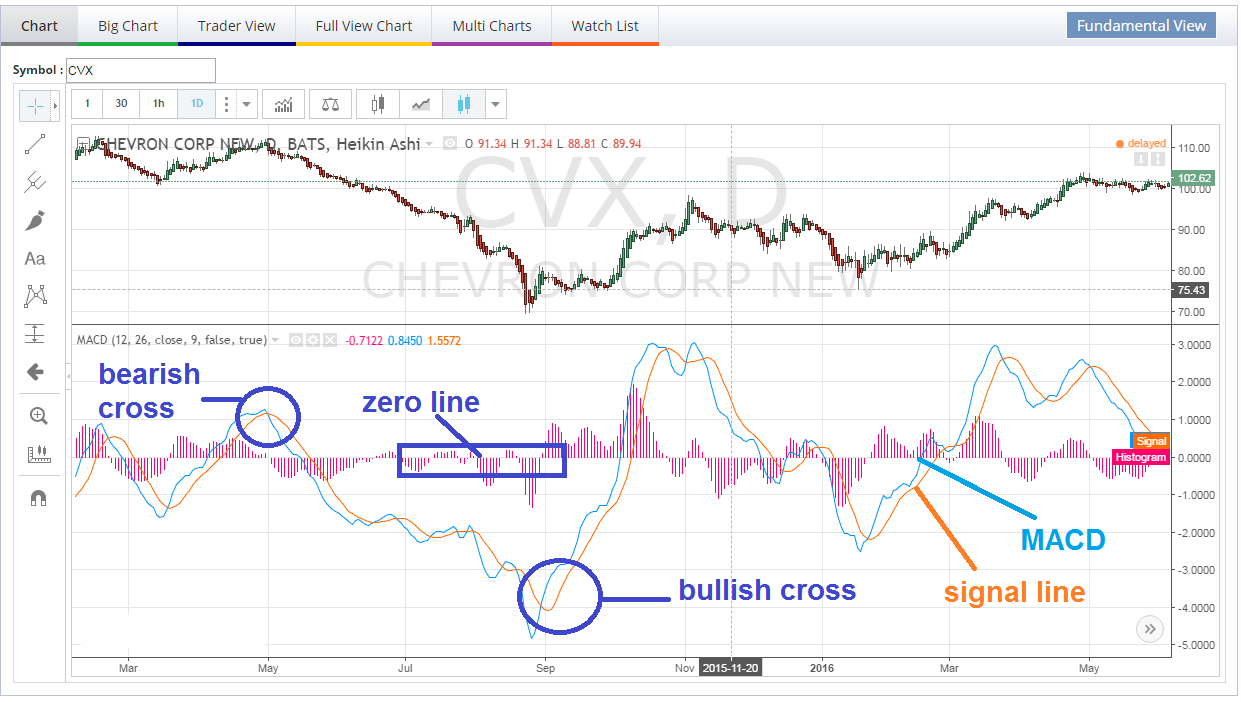# What is the MACD Indicator and is it worth using?

## What is the ‘Moving Average Convergence Divergence – MACD’

Moving average convergence divergence (MACD) is a trend-following momentum indicator that shows the relationship between two moving averages of prices. The MACD is calculated by subtracting the 26-day exponential moving average (EMA) from the 12-day EMA. A nine-day EMA of the MACD, called the “signal line”, is then plotted on top of the MACD, functioning as a trigger for buy and sell signals.

Investors like use the MACD because it is suppose to smooth out the price volatility and generate signals with bullish and bearish crossovers below.  After extensive back testing using the MACD, it does not provide high probability outcomes. The main reason this indicator is not that great is because its not robust and confirmation of momentum longer trend trends is weak. Additionally, this is not an indicator for intermediate to longer term trend followers as it generates too many false signals.  We rarely usie it for longer term sell signals because momentum can trend while the MACD illustrates decreasing momentum and generates a bearish crossover.

The one way we do use the MACD on a daily, weekly or even a 5 or 30 minute chart is for possible counter trend oversold rallies. (see counter trends).  We look for a very deep retracement of the MACD and signal line well below the zero line with an RSI below 30.  Once the Signal line Crosses the MACD still below the Zero line.1. Crossovers –

• As shown in the chart above, when the MACD falls below the signal line, it is a bearish signal.
• Conversely, when the MACD rises above the signal line, the indicator gives a bullish signal. Additionally, waiting for both the MACD / Signal line to cross above zero line.